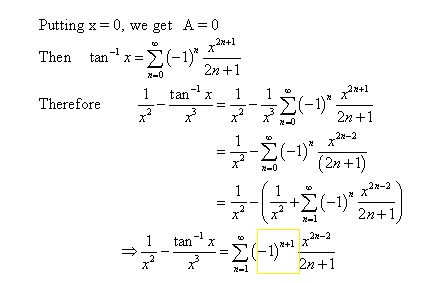# Function represented as a power series

## Homework Statement

[url]http://img.photobucket.com/albums/v257/gamer567/powerseries.png[/url]

## The Attempt at a Solution

[url]http://img.photobucket.com/albums/v257/gamer567/cramster.png[/url]

I am getting lost from the transition in the 1/x-2 to 1/(x-2)^2. Could someone explain to me what happened? I think I could finish this problem and several others if I could understand this. Also is there a better approach? In other problems I took the derivative and put into a power series and then took the integral. This doesn't seem to be working on this one(?)

Apologies I am unable to edit, the equation in the picture needs to be represented as a power series if I didn't make it clear.

Before differentiating, the right-hand side can be rewritten as

$$\frac{-1}{2} \sum \frac{x^n}{2^n} = - \sum \frac{x^n}{2^{n+1}}$$

Thus, we differentiate to get:

$$\frac{d}{dx}[\frac{1}{x-2}] = \frac{d}{dx} [ - \sum \frac{x^n}{2^{n+1}}]$$

$$\frac{-1}{(x-2)^2} = - \sum \frac{n}{2^{n+1}}x^{n-1}$$

The reason we do this is because now we can multiply both sides by -x to give us:

$$\frac{x}{(x-2)^2} = \sum \frac{n}{2^{n+1}}x^{n}.$$

Do you see how this is helpful in trying to get to the power series of f(x)?

Also is there a better approach? In other problems I took the derivative and put into a power series and then took the integral. This doesn't seem to be working on this one(?)

As far as I know, this is the standard and easiest approach. You should learn and understand this method because I wouldn't be surprised if it pops up in some other problems.

As far as I know, this is the standard and easiest approach. You should learn and understand this method because I wouldn't be surprised if it pops up in some other problems.

Awesome that helps :) Got confused when they rearranged stuff while saying they are taking derivative.

No doubt this would be a nice mean test question :( Shall be redoing this one a couple times.^^ New problem

If you dont mind me asking one more question, I am stuck as to how they got the last line of this solution to another problem.

From what I can tell, they plug in n=0 and got 1/(x^2) and then changed the power series to n=1

1/(x^2)-1/(x^2) cancels out and disappears. The thing I don't understand is how (-1)^n becomes (-1)^(n+1)

What am I missing here? The answer in the back of the book has it as well.

There's a negative sign in front of $$tan^{-1}x.$$ They bring it into its power series formula, thus raising the exponent on -1 to n + 1.